# Shortest Path Algorithm Dijkstras Algorithm BellmanFord Algorithm 8

• Slides: 17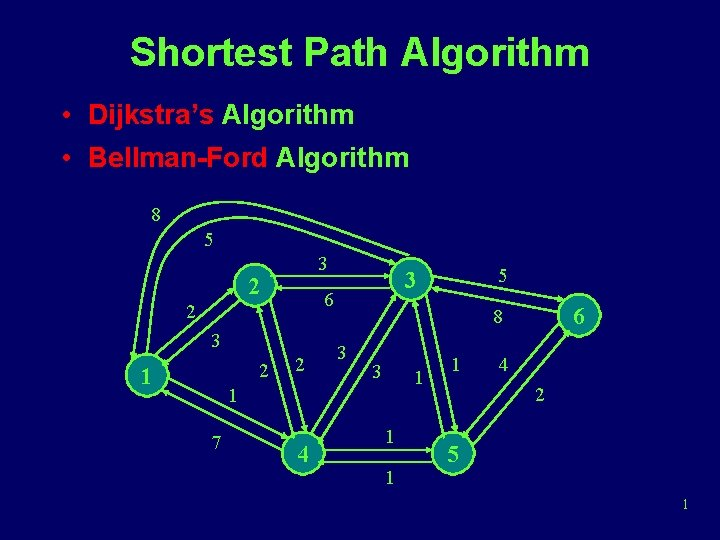Shortest Path Algorithm • Dijkstra’s Algorithm • Bellman-Ford Algorithm 8 5 3 2 6 2 3 2 1 2 4 6 8 3 3 1 1 7 5 3 1 1 1 4 2 5 1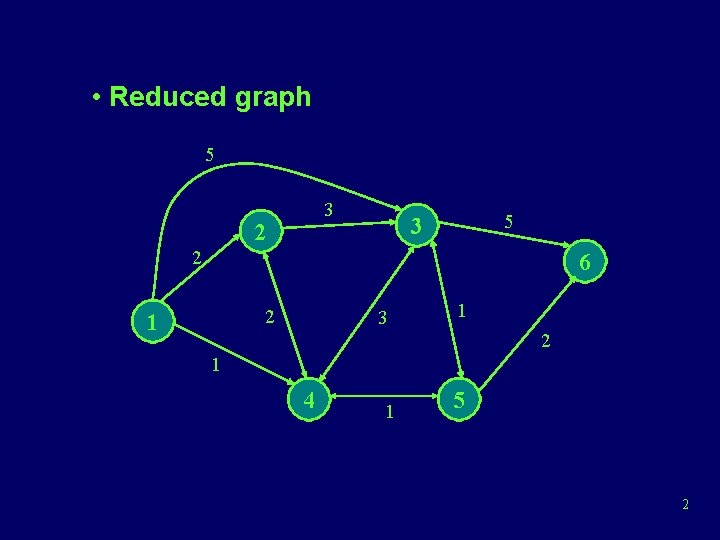• Reduced graph 5 3 2 6 2 1 3 1 2 1 4 1 5 2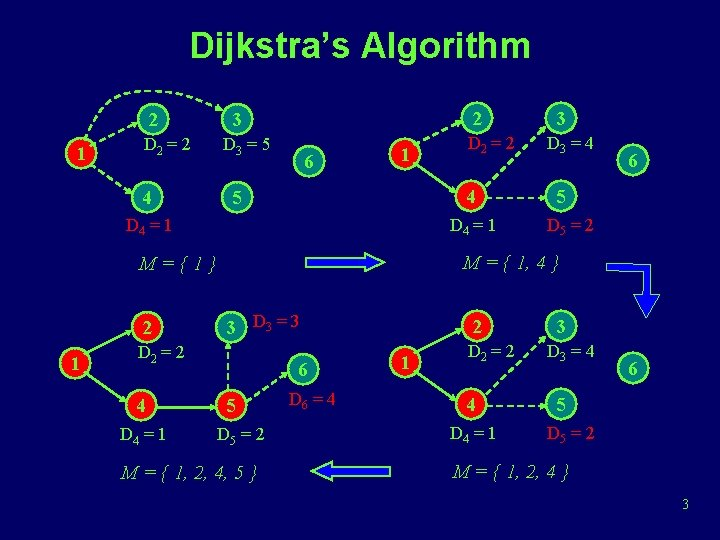Dijkstra’s Algorithm 2 1 D 2 = 2 4 2 3 D 3 = 5 6 1 4 5 D 4 = 1 1 3 D 3 = 3 D 2 = 2 4 D 4 = 1 D 3 = 4 6 5 D 5 = 2 M = { 1, 4 } M={1} 2 D 2 = 2 3 6 5 D 5 = 2 M = { 1, 2, 4, 5 } D 6 = 4 2 1 D 2 = 2 4 D 4 = 1 3 D 3 = 4 6 5 D 5 = 2 M = { 1, 2, 4 } 3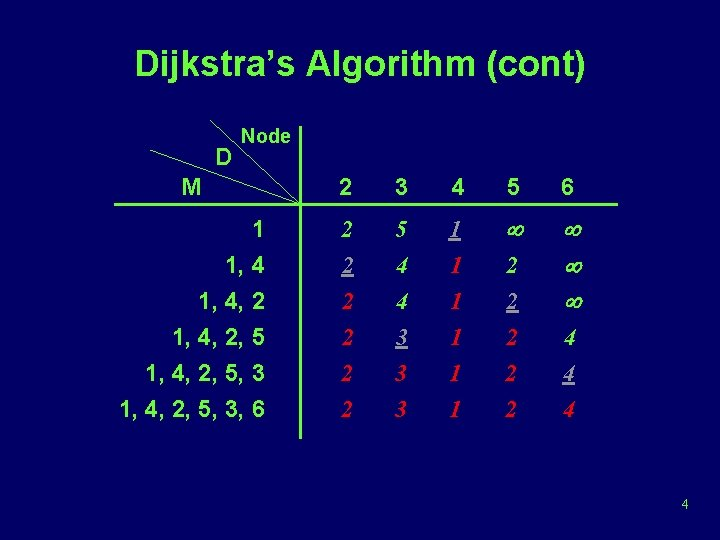Dijkstra’s Algorithm (cont) D Node M 2 3 4 5 6 1 1, 4, 2, 5, 3 1, 4, 2, 5, 3, 6 2 5 1 ¥ 2 2 2 4 4 3 3 3 1 1 1 2 2 2 ¥ ¥ ¥ 4 4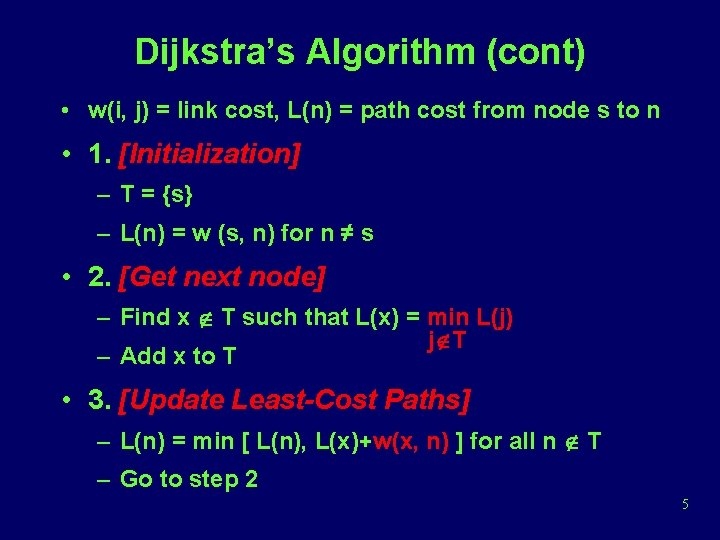Dijkstra’s Algorithm (cont) • w(i, j) = link cost, L(n) = path cost from node s to n • 1. [Initialization] – T = {s} – L(n) = w (s, n) for n ≠ s • 2. [Get next node] – Find x Ï T such that L(x) = min L(j) jÏT – Add x to T • 3. [Update Least-Cost Paths] – L(n) = min [ L(n), L(x)+w(x, n) ] for all n Ï T – Go to step 2 5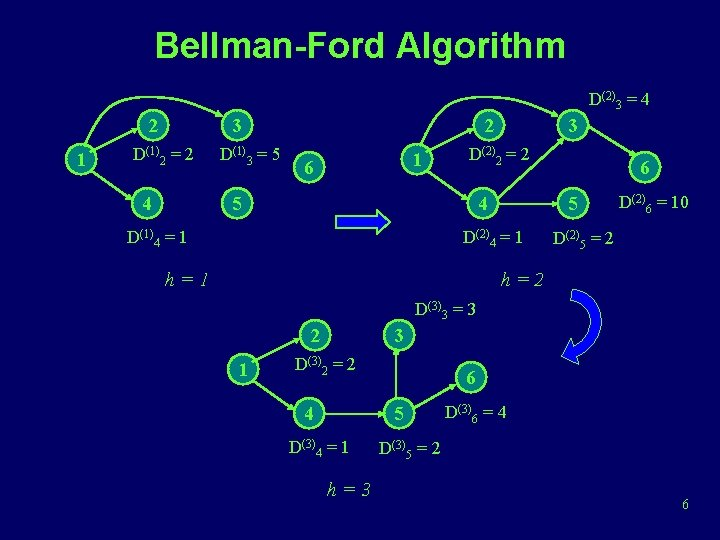Bellman-Ford Algorithm D(2)3 = 4 2 1 3 D(1)2 = 2 4 D(1)3 = 5 2 1 6 3 D(2)2 = 2 5 4 D(1)4 = 1 5 D(2)4 = 1 h=1 6 D(2)6 = 10 D(2)5 = 2 h=2 D(3)3 = 3 2 1 3 D(3)2 = 2 4 6 5 D(3)4 = 1 h=3 D(3)6 = 4 D(3)5 = 2 6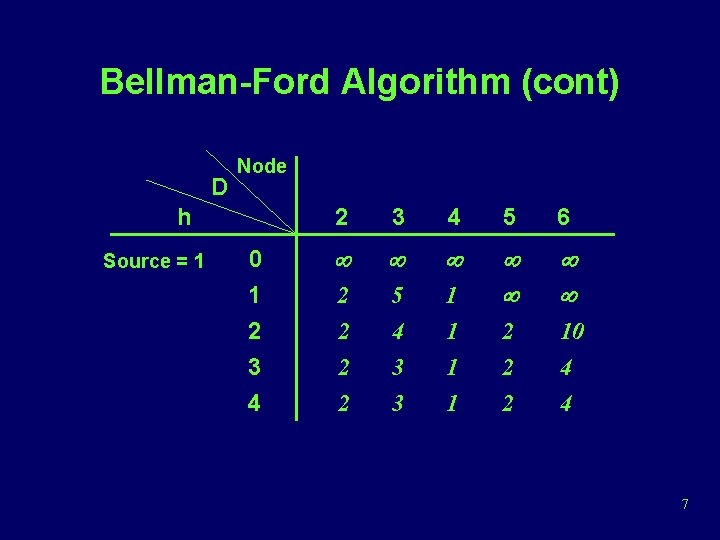Bellman-Ford Algorithm (cont) D Node h Source = 1 0 1 2 3 4 5 6 ¥ ¥ ¥ 2 5 1 ¥ ¥ 2 2 2 4 3 3 1 1 1 2 2 2 10 4 4 7Bellman-Ford Algorithm (cont) • Lh(n) = path cost from s to n w/ no more than h links • 1. [Initialization] – L 0(n) = ∞, for all n ≠ s – Lh(s) = 0, for all h link j s • 2. [Update] – For each successive h ≥ 0 n <= h links – For each n ≠ s, compute – Lh+1(n) = min [ Lh(j) + w(j, n) ] j 8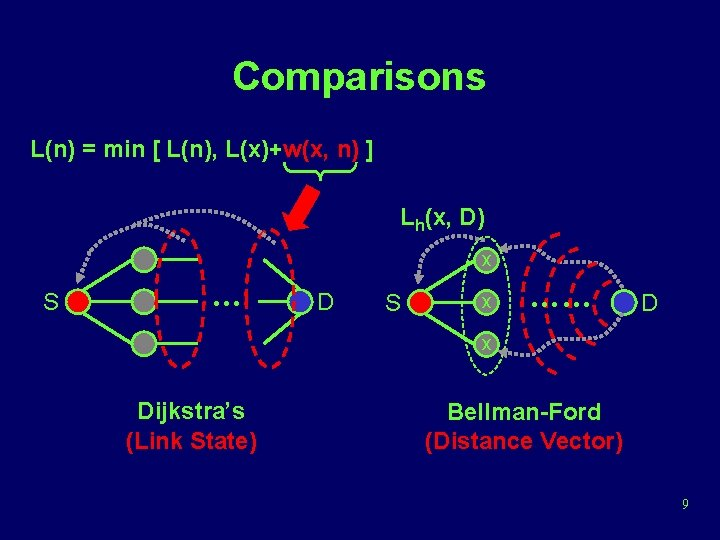Comparisons L(n) = min [ L(n), L(x)+w(x, n) ] Lh(x, D) x S … D S x …… D x Dijkstra’s (Link State) Bellman-Ford (Distance Vector) 9Routing in ARPANET • First generation(RIP), 1969 – Adaptive Routing is adopted – Use Bellman-Ford algorithm – Estimated link delay is simply the queue length for that link – Every 128 ms, each node exchanges its delay vector(routing table) with all its neighbors – Information about a change in network condition would gradually ripple through the network 10Routing in ARPANET (cont) – Each node i maintains • di j = current estimate of min delay from i to j • si j = next node in the current min-delay route from i to j – Node k updates its vectors as follows • dk j = Min [ lk i + di j ] iÎA • sk j = i k i j using i that minimizes the expression above where A = set of neighbor nodes for k lk i = current estimate of delay from k to i 11Routing in ARPANET (cont) • Major shortcomings of RIP – It did not consider line speed, merely queue length. Higher capacity links were not given the favored status – Queue length is an artificial measure of delay – The algorithm was not very accurate. It responded slowly to congestion and delay increases. 12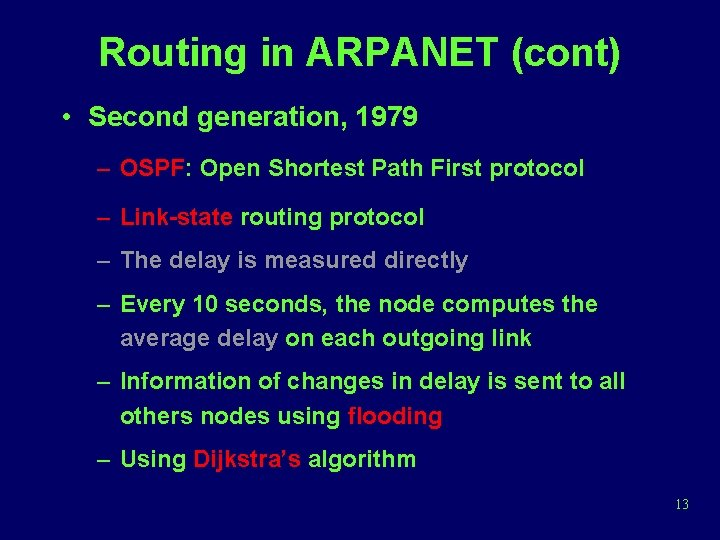Routing in ARPANET (cont) • Second generation, 1979 – OSPF: Open Shortest Path First protocol – Link-state routing protocol – The delay is measured directly – Every 10 seconds, the node computes the average delay on each outgoing link – Information of changes in delay is sent to all others nodes using flooding – Using Dijkstra’s algorithm 13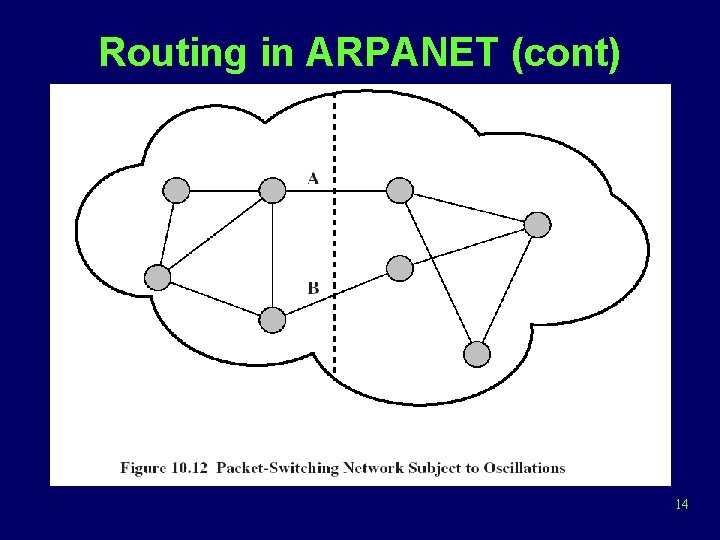Routing in ARPANET (cont) 14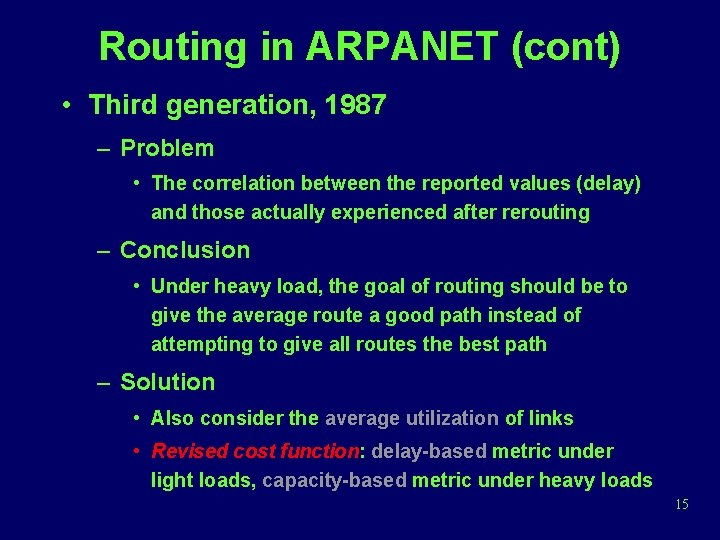Routing in ARPANET (cont) • Third generation, 1987 – Problem • The correlation between the reported values (delay) and those actually experienced after rerouting – Conclusion • Under heavy load, the goal of routing should be to give the average route a good path instead of attempting to give all routes the best path – Solution • Also consider the average utilization of links • Revised cost function: delay-based metric under light loads, capacity-based metric under heavy loads 15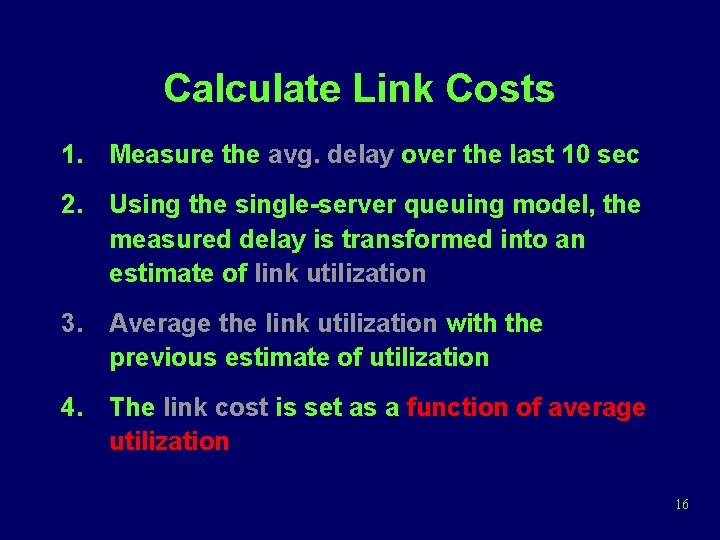Calculate Link Costs 1. Measure the avg. delay over the last 10 sec 2. Using the single-server queuing model, the measured delay is transformed into an estimate of link utilization 3. Average the link utilization with the previous estimate of utilization 4. The link cost is set as a function of average utilization 16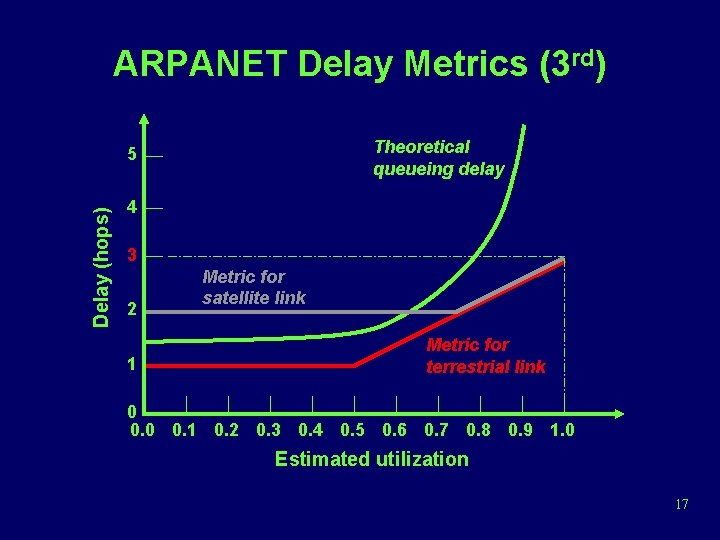ARPANET Delay Metrics (3 rd) Theoretical queueing delay Delay (hops) 5 4 3 2 1 Metric for satellite link Metric for terrestrial link 0 0. 1 0. 2 0. 3 0. 4 0. 5 0. 6 0. 7 0. 8 0. 9 1. 0 Estimated utilization 17